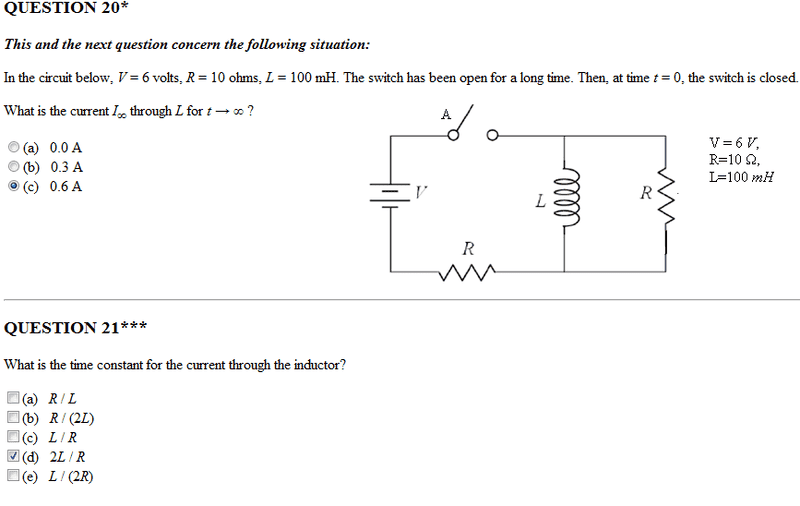# Time constant for an inductor?

The original question:Notice that they have marked that the correct time constant is T = 2L/R

Why is this the correct answer and not L/R? I thought the inductor will only take into consideration one Resistor as in both circuits (switch closed vs open), current will only flow through one resistor at a time.

EDIT: Moderator's note -- I have moved this thread from Advanced Physics to Intro Physics -- Redbelly98

Last edited by a moderator:

Hi...!
Have you tried using Ohm's law, and solving for the currents?
It seems that one can obtain the right answer by doing that; Recall that the voltage that is exerted on both the Inductor and the second resistor is not V but V-I*R, and thus you should obtain the proper image, I'd think.
Daniel

gneill
Mentor
For Question 21 they really should have specified whether the switch is open or closed. The circuit will have a different time constant for each position.

When the switch is open the inductor "sees" only one resistor, the rightmost one. So the time constant is L/R. But when the switch is closed it "sees" both resistors. Until the circuit reaches steady-state after a long time, currents will be flowing in both resistors (Eventually the inductor looks like a short circuit current flows only through the lower-left R).

In order to calculate the time constant for the switch-closed case you will have to determine what total resistance the inductor "sees". To do that, remove the inductor from the circuit and suppress any sources (in this case you short the battery). Determine the equivalent resistance of the resulting network looking into the inductor's connection points.

https://www.physicsforums.com/attachment.php?attachmentid=41329&stc=1&d=1322484717